Test: Matrices

# Test: Matrices

Test Description

## 10 Questions MCQ Test Engineering Mathematics | Test: Matrices

Test: Matrices for Civil Engineering (CE) 2023 is part of Engineering Mathematics preparation. The Test: Matrices questions and answers have been prepared according to the Civil Engineering (CE) exam syllabus.The Test: Matrices MCQs are made for Civil Engineering (CE) 2023 Exam. Find important definitions, questions, notes, meanings, examples, exercises, MCQs and online tests for Test: Matrices below.
Solutions of Test: Matrices questions in English are available as part of our Engineering Mathematics for Civil Engineering (CE) & Test: Matrices solutions in Hindi for Engineering Mathematics course. Download more important topics, notes, lectures and mock test series for Civil Engineering (CE) Exam by signing up for free. Attempt Test: Matrices | 10 questions in 30 minutes | Mock test for Civil Engineering (CE) preparation | Free important questions MCQ to study Engineering Mathematics for Civil Engineering (CE) Exam | Download free PDF with solutions
 1 Crore+ students have signed up on EduRev. Have you?
Test: Matrices - Question 1

### Comprehension: Direction: Based on the following information, answer the questions: Abhi, Badri, and Chintan were given the task of creating a square matrix of order 3. Below are the matrices created by them. A, B, and C are the matrices created by Abhi, Badri, and Chintan respectively.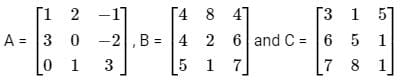Let matrix B = P + Q, where P is a symmetric matrix and Q is a skew - symmetric matrix. What is Q?

Detailed Solution for Test: Matrices - Question 1

For any matrix A, (A + A’) is always symmetric and (A - A') is always skew-symmetric.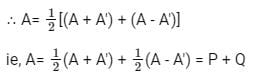where P = 1/2(A+ A'), a symmetric matrix and Q = 1/212(A - A'), is a skew-symmetric matrix.

Caluclation: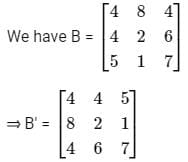∴ Required symmetric matrix Q
= 1/2 (B-B')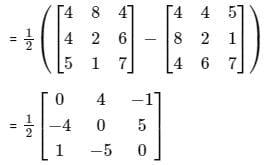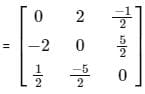Test: Matrices - Question 2

### Direction: Based on the following information, answer the questions: Abhi, Badri, and Chintan were given the task of creating a square matrix of order 3. Below are the matrices created by them. A, B, and C are the matrices created by Abhi, Badri, and Chintan respectively.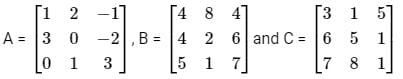What is (AT)T

Detailed Solution for Test: Matrices - Question 2

If A is any matrix, the (AT)T = A.

We have,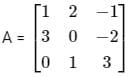∴ (AT)T

= A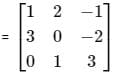Test: Matrices - Question 3

### If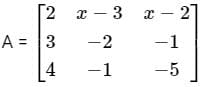is a symmetric matrix then x

Detailed Solution for Test: Matrices - Question 3

Given:
A is a symmetric matrix,
⇒ AT = A or aij = aji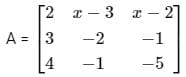So, by property of symmetric matrices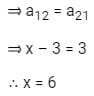Test: Matrices - Question 4

lf the order of A is 4 × 3, the order of B is 4 × 5 and the order of C is 7 × 3, then the order of (ATB)T CT is

Detailed Solution for Test: Matrices - Question 4

Given:
Order of A is 4 × 3, the order of B is 4 × 5 and the order of C is 7 x 3
The transpose of the matrix obtained by interchanging the rows and columns of the original matrix.
So, order of AT is 3 × 4 and order of CT is 3 × 7
Now,
ATB = {3 x 4} {4 × 5} = 3 x 5
⇒ Order of ATB is 3 x 5
Hence order of (ATB)T is 5 x 3
Now order of (ATB)T CT = {5 x 3} {3 x 7} = 5 x 7
∴ Order of (ATB)T CT is 5 x 7

Test: Matrices - Question 5

If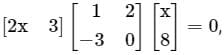then the value of x is

Detailed Solution for Test: Matrices - Question 5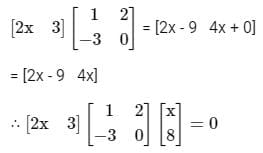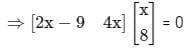⇒ [(2x - 9)x + 8 x 4x] = 0

⇒ [2x2 - 9x + 32x] = 0

⇒ 2x2 + 23x = 0

⇒ x(2x + 23) = 0

⇒ x = 0 or - 23/2

Test: Matrices - Question 6

If A is an Involuntary matrix and I is a unit matrix of same order, then (I − A) (I + A) is

Detailed Solution for Test: Matrices - Question 6

Given that A is involuntary matrix,
⇒ A2 = I
Now,
(I − A) (I + A) = I2 – IA + AI − A2
⇒ I – A + A – I         (∵ A2 = I)
⇒ 0
∴ (I − A) (I + A) is zero matrix.

Test: Matrices - Question 7

Consider the following question and decide which of the statements is sufficient to answer the question.
Find the value of n, if
Statements∶
1. AB = A
2.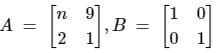Detailed Solution for Test: Matrices - Question 7

From statement 1∶
AB = A
We cannot find anything from this statement.
From statement 2∶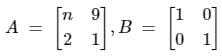We cannot find anything from this statement.
Combining statement 1 and 2∶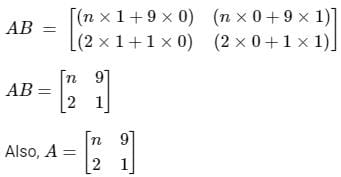∴ We cannot find the value of n from both statements together.

Test: Matrices - Question 8

If A2 - 2A - I = 0,then inverse of A is

Detailed Solution for Test: Matrices - Question 8

Given: A2 - 2A - I = 0
⇒ A.A - 2A = I
Post multiply by A-1, we get
⇒ AAA-1 - 2AA-1 = IA-1
⇒ AI - 2I = A-1             [∵ AA -1 = A - 1A = I]
∴ A-1 = A - 2
the inverse of A is A - 2

Test: Matrices - Question 9

If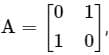then the value of A4 is

Detailed Solution for Test: Matrices - Question 9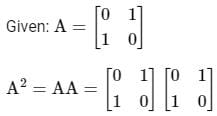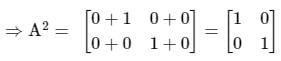Now,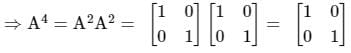Hence Option 1st is correct answer.

Test: Matrices - Question 10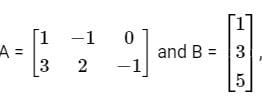find (AB)T

Detailed Solution for Test: Matrices - Question 10

Given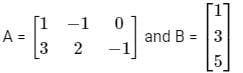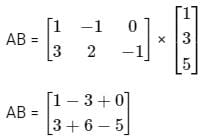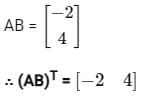## Engineering Mathematics

47 videos|119 docs|75 tests(Scan QR code)